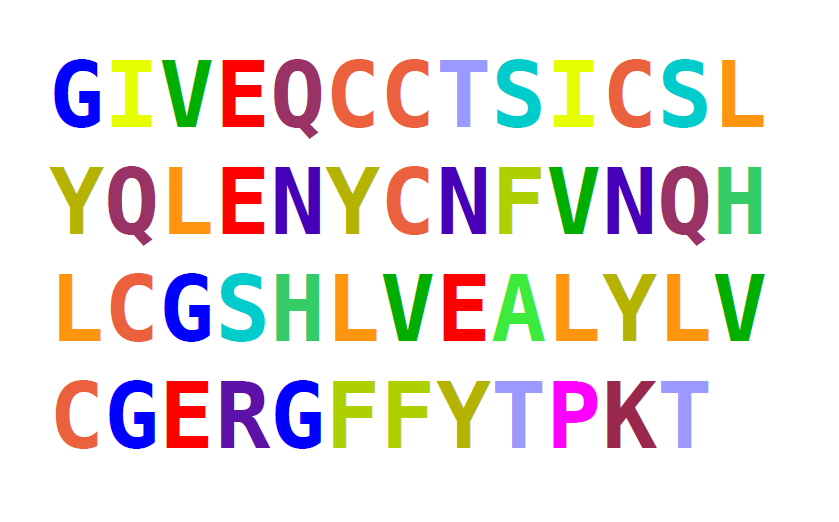# Making decisions

The last missing piece in our basic set of commands is the ability to make decisions in a program. This is done in Python using the `if` command.### Exercise 1

Execute the following program and explain its output.

``````number = 123

if number > 1000:
print("The number is larger than 1000.")
elif number == 1000:
print("The numer is exactly 1000.")
else:
print("The number is smaller than 1000.")
``````

### Exercise 2

Set `name` to such a value that one, two or all three conditions apply.

``````name = ____

if "m" in name:
print("There is a 'm' in the name.")
if name != "Mimi":
print("The name is not Mimi.")
if name == "M" and name[-1] == "m":
print("The name starts and ends with m.")
``````

### Exercise 3

The following program writes the positions of all letters "n" in the name to the screen. Unfortunately, it contains three errors. Make the program execute correctly:

``````name = "Anna"
position = 1

for char in name
if char = "n":
print(position)
position = position + 1
``````

### Exercise 4

Which of these `if` statements are syntactically correct?

• `if a and b:`
• `if len(s) == 23:`
• `if a but not b < 3:`
• `if a ** 2 >= 49:`
• `if a != 3`
• `if (a and b) or (c and d):`